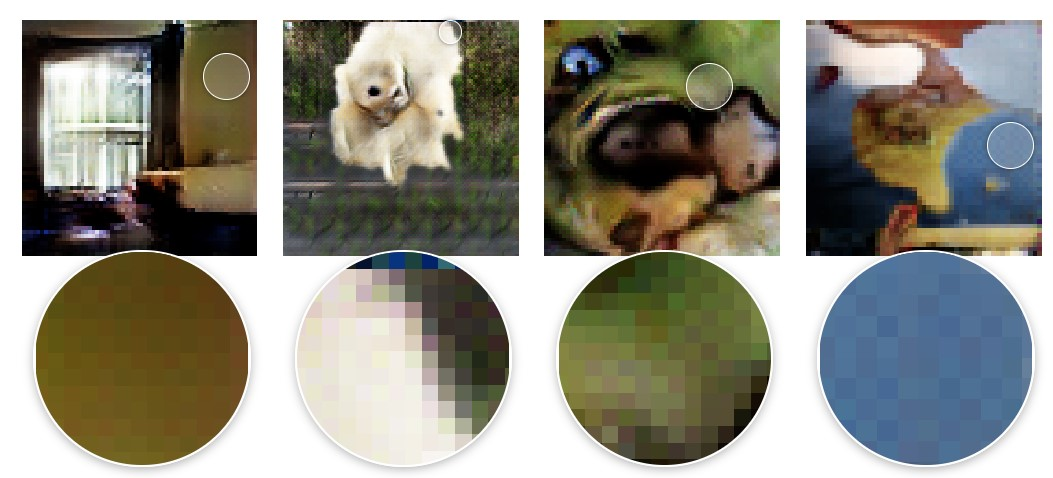×

Search anything:

# Transposed Convolution

#### Machine Learning (ML) Deep LearningGet this book -> Problems on Array: For Interviews and Competitive Programming

# Introduction:

Convolutional neural networks are very useful when it comes to computer vision projects and can be automatically used to extract features from photos and videos while also reducing the image dimensions.
In most models for image classification, the CNN that is used is with a fully-connected layer. But in this case there are several issues that you will face like higher computation cost and no preservation of the spatial dimensions. To solve this problem, we employ a technique called Transpose Convolution, which is just the inverse of a standard convolution. This is accomplished by keeping the connectivity pattern intact.

# Definition:

Transposed convolution is also known as upsampled convolution, which refers to the task it accomplishes, which is to upsample the input feature map.
Because stride over the output is equal to fractional stride over the input, it's also known as fractionally strided convolution.
Since the forward pass of a Transposed Convolution is the same as the backward pass of a normal convolution, it is called backward strided convolution.

Code:
Implementation of transposed convolution-
Input shape:
3D tensor with shape: (batchsize, steps, channels)
Output shape:
3D tensor with shape: (batchsize, newsteps, filters)

For example -

``````tf.keras.layers.Conv1DTranspose(
filters,
kernel_size,
strides=1,
dilation_rate=1,
activation=None,
use_bias=True,
kernel_initializer='glorot_uniform',
bias_initializer='zeros
)
``````

# What is the goal?

The goal in image classification is to get the output image with the same dimensions without running into the issues faced by a fully Convolutional Neural Network. To achieve this we have to enforce the upsampling of the input so that it matches the output dimensions.

# Commonly used upsampling techniques:

Nearest Neighbors:
Nearest Neighbors takes an input pixel value and copies it to the K-Nearest Neighbors, where K is the expected output.
Bi-Linear Interpolation:
In this, we take the four closest pixel values of the input pixel and smoothen the output using a weighted average based on the distance between the four closest cells.
Bed of Nails:
In Bed of Nails, we replicate the value of the input pixel to the output image's corresponding place while filling the rest positions with zeros.
Max-Unpooling:
The Max-Pooling layer selects the highest value from all the values in the kernel. To accomplish max-unpooling, each max-pooling layer's index of the maximum value is saved throughout the encoding step. The saved index is subsequently used in the decoding step, where the input pixel is mapped to the saved index, with zeros filling in the blanks.

And then coming to transposed convolution another way of upsampling which has an added feature of using some learnable parameters. It does not rely on a pre-programmed interpolation mechanism.

# Transposed Convolution-

The steps involved:

1. Assume you have a 2x2 input that needs to be upsampled to a 3x3 output.
2. Next, take a kernel of size 2x2 with unit stride and zero padding.
3. Then the next step involves taking the upper left element of the input and to multiply it with every element of the kernel.
4. We repeat this process for all of the remaining input components.This forms four different 2x2 matrices for each of the elements of the input and these are mapped according to their position.
5. As they are mapped according to their position, some of the elements of the resulting upsampled matrices will cause over-lapping. We just add the elements of the over-lapping places to fix this problem.
6. The resulting output will be the final upsampled matrix having the required spatial dimensions of 3x3.

Even though it's named the transposed convolution, that doesn't mean we utilise the transposed version of an existing convolution matrix. The important feature is that, in contrast to a standard convolution matrix, the relationship between the input and the output is handled backwards (one-to-many rather than many-to-one association).

As a result, the transposed convolution isn't one at all. However, we can use a convolution to simulate the transposed convolution.

Transposed convolutions can easily have strange checkerboard patterns as shown below.The fundamental reason for this is that some sections of the image have unequal overlap, resulting in artefacts. One way to avoid the overlap issue is to make sure you use a kernel size that is divided by your stride. Thus by using a kernel size that is divisible by the stride, such as 2x2 or 4x4 when the stride is 2.

# Conclusion-

Modern image-segmentation and super-resolution algorithms are built on the foundation of transposed convolutions. They offer the most accurate and comprehensive upsampling. We looked at the various commonly used upsampling techniques, steps of transposed convolution, disadvantages of transposed convolutions and then finally the applications of transposed convolutions.